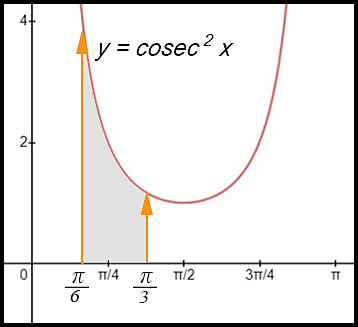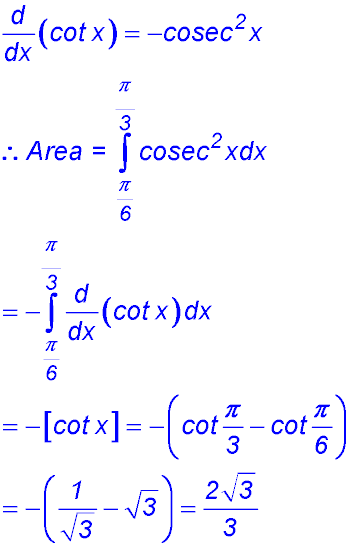Dr. J's Maths.com
Where the techniques of Maths
are explained in simple terms.

Trigonometric functions - Calculus - Differentiate ... hence find.
Test Yourself 1 - Solutions.

 1. (i)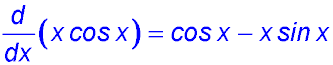(ii)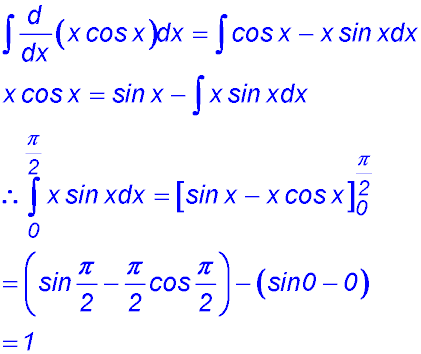2. (i)(ii)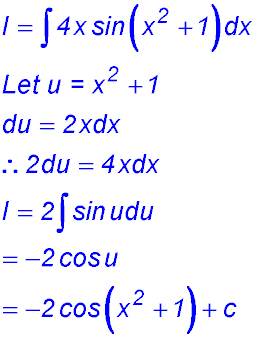3. (i)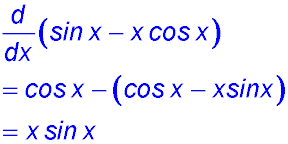(ii)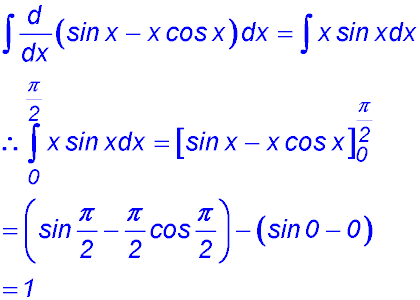4.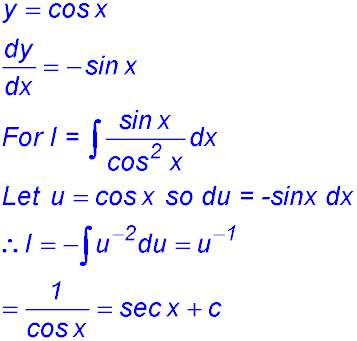5. 7.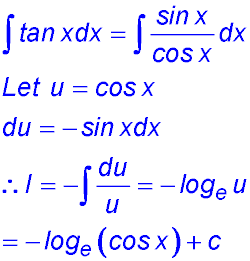8. (i)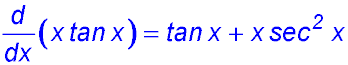. (ii)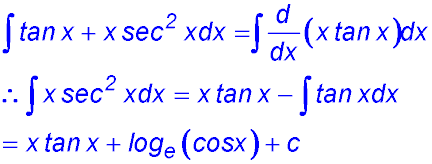9. (i)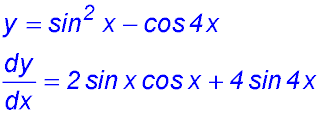(ii)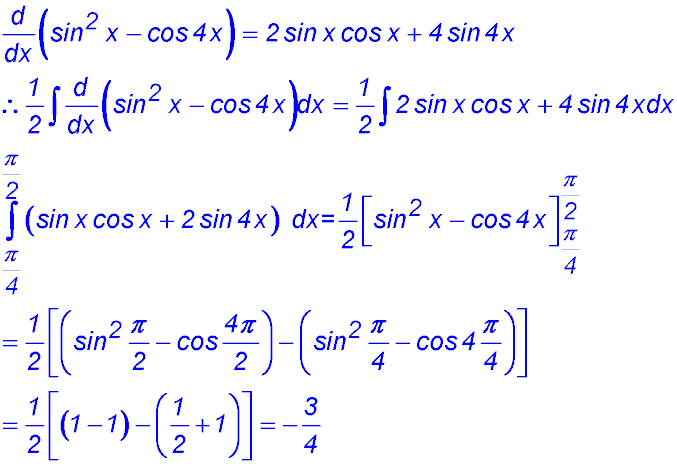10. (i)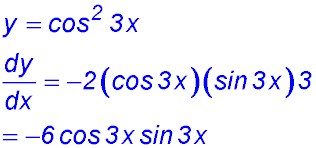. (ii)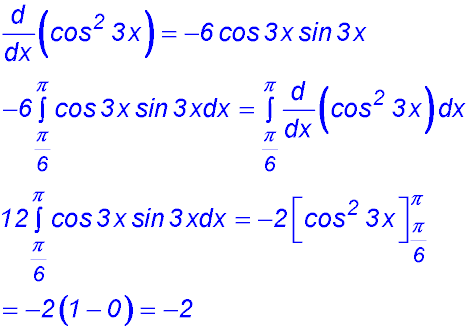. 11. 12. 13. (i)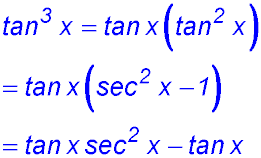(ii)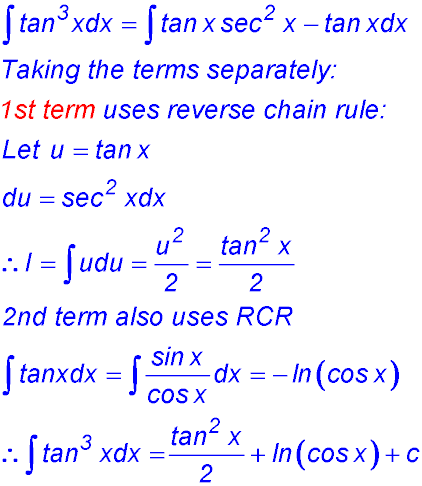14. 15.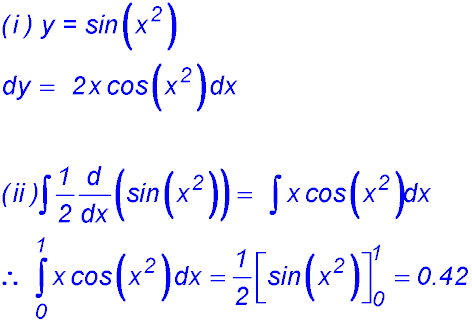16. (i)(ii)